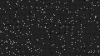# Pixel Flipboard Style Animation

#### tagwolf

##### Member
GM Version: GMS2
Target Platform: ALL

Summary:
Ever wanted to make cool pixel flipboard style animations? Well now you can. If you want it to actually draw something you could do so easy enough by making a collision mask of your images and then have that change the enable variable on the one's it's touching. You could even make your whole game use flipboard style pixel animations this way. Right now the project is set to do it randomly with random delays (all configurable). Even if you don't use it, you could export it and change the EXE to an SCR file and it makes a cool screensaver IMO.

Tutorial:
See project file! (https://host-a.net/f/226893-flipboard-animationyyz)create
Code:
``````/// @description Init Vars
image_yscale = -1;
inverted = true;
flip_rate = 0.05;
flip_it = true;
enabled = true;
max_delay = 1000;

light_alpha = random_range(0.80,1.00);
dark_alpha = random_range(0.15,0.20);

//enabled = irandom(1);
delay = irandom(max_delay);

//image_blend = choose(c_red, c_green, c_blue);``````
step
Code:
``````/// @description Rotate / Flip Pixel

//show_debug_message(string(image_yscale));
//show_debug_message(string(flip_it));
//show_debug_message(string(inverted));

if image_yscale > 0 { image_alpha = light_alpha; }
if image_yscale < 0 { image_alpha = dark_alpha; }

if enabled {
if flip_it
{
if delay <= 0
{
if image_yscale >= -1 && inverted == false && flip_it
{
image_yscale -= flip_rate;
if image_yscale - flip_rate <= -1 || image_yscale <= -1
{
//flip_it = false;
inverted = true;
image_yscale = -1;
delay = irandom(max_delay);
}
}

if image_yscale <= 1 && inverted == true && flip_it
{
image_yscale += flip_rate;
if image_yscale + flip_rate >= 1 || image_yscale >= 1
{
//flip_it = false;
inverted = false;
image_yscale = 1;
delay = irandom(max_delay);
}
}
}
else
{
delay--;
}
}
}``````

Last edited: